11.6: Rates of Reactions

•• Contributed by Allison Soult
• Senior Lecturer (Chemistry) at University of Kentucky

Learning Outcomes

• Define reaction rate.
• Define catalyst and explain its behavior in a chemical reaction.
• Describe how temperatures, concentration or pressure of reactant, and a catalyst affect the reaction rate.
• Explain the concept of activation energy.
• Label a diagram with reactants, products, enthalpy of forward and reverse reactions, activation energy of forward and reverse reactions, and activated complex.
• Calculate the enthalpy and activation energy with data on a reaction diagram.

Chemical kinetics is the study of the rates of chemical reactions. In this lesson, you will learn how to express the rate of a chemical reaction and about various factors that influence reaction rates.

Expressing Reaction Rate

Chemical reactions vary widely in the speeds with which they occur. Some reactions occur very quickly. It a lighted match is brought into contact with lighter fluid or another flammable liquid, it erupts into flame instantly and burns fast. Other reactions occur very slowly. A container of milk in the refrigerator will be good to drink for weeks before it begins to turn sour. Millions of years were required for dead plants under Earth's surface to accumulate and eventually turn into fossil fuels such as coal and oil.

Chemists need to be concerned with the rates at which chemical reactions occur. Rate is another word for speed. If a sprinter takes 11.0 seconds $$\left( s \right)$$ to run a 100 meter (\left( m \right)\) dash, his rate or speed is given by the distance traveled divided by the time (see figure below).

$\text{speed} = \frac{\text{distance}}{\text{time}} = \frac{100 \: \text{m}}{11.0 \: \text{s}} = 9.09 \: \text{m/s}$

The sprinter's average running rate for the race is $$9.09 \: \text{m/s}$$. We say that it is his average rate because he did not run at that speed for the entire race. At the very beginning of the race, while coming from a standstill, his rate must be slower until he is able to get up to his top speed. His top speed must the be greater than $$9.09 \: \text{m/s}$$ so that taken over the entire race, the average ends up at $$9.09 \: \text{m/s}$$.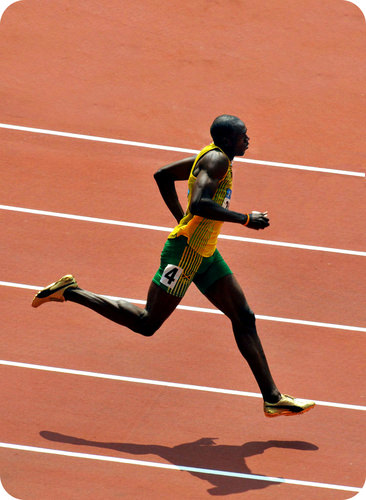Figure $$\PageIndex{1}$$: Usain Bolt set the world record for the 100 meter dash in 2009 with a time of 9.58 seconds. His average running rate over the course of this race was $$10.4 \: \text{m/s}$$, or $$23.4 \: \text{mph}$$.

Chemical reactions can't be measured in units of meters per second, as that would not make any sense. A reaction rate is the change in concentration of a reactant or product with time. Suppose that a simple reaction were to take place in which a 1.00 molar $$\left( \text{M} \right)$$ aqueous solution of substance $$\ce{A}$$ was converted to substance $$\ce{B}$$.

$\ce{A} \left( aq \right) \rightarrow \ce{B} \left( aq \right)$

Suppose that after 20.0 seconds, the concentration of $$\ce{A}$$ had dropped from $$1.00 \: \text{M}$$ to $$0.72 \: \text{M}$$ as it was being converted to substance $$\ce{B}$$. We can express the rate of this reaction as the change in concentration of $$\ce{A}$$ divided by the time.

$\text{rate} = -\frac{\Delta \left[ \ce{A} \right]}{\Delta t} = -\frac{\left[ \ce{A} \right]_\text{final} - \left[ \ce{A} \right]_\text{initial}}{\Delta t}$

A bracket around a symbol or formula means the concentration in molarity of that substance. The change in concentration of $$\ce{A}$$ is its final concentration minus its initial concentration. Because the concentration of $$\ce{A}$$ is decreasing over time, the negative sign is used. Thus, the rate for the reaction is positive, and the units are molarity per second or $$\text{M/s}$$.

$\text{rate} = -\frac{0.72 \: \text{M} - 1.00 \: \text{M}}{20.0 \: \text{s}} = -\frac{-0.28 \: \text{M}}{20.0 \: \text{s}} = 0.041 \: \text{M/s}$

Over the first 20.0 seconds of this reaction, the molarity of $$\ce{A}$$ decreases by an average rate of $$0.041 \: \text{M}$$ every second. In summary, the rate of a chemical reaction is measured by the change in concentration over time for a reactant or product. The unit of measurement for a reaction rate is molarity per second $$\left( \text{M/s} \right)$$.

Collision Theory

The behavior of the reactant atoms, molecules, or ions is responsible for the rates of a given chemical reaction. Collision theory is a set of principles based around the idea that reactant particles form products when they collide with one another, but only when those collisions have enough kinetic energy and the correct orientation to cause a reaction. Particles that lack the necessary kinetic energy may collide, but the particles will simply bounce off one another unchanged. The figure below illustrates the difference. In the first collision, the particles bounce off one another, and no rearrangement of atoms has occurred. The second collision occurs with greater kinetic energy, and so the bond between the two red atoms breaks. One red atom bonds with the other molecule as one product, while the single red atom is the other produce. The first collision is called an ineffective collision, while the second collision is called an effective collision.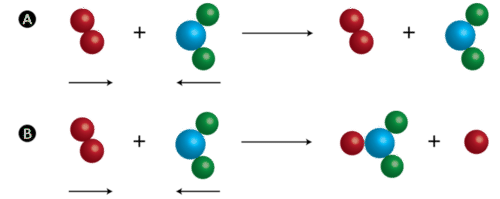Figure $$\PageIndex{2}$$: (A) An ineffective collision is one that does not result in product formation. (B) An effective collision is one in which chemical bonds are broken, and a product is formed.

Supplying reactant particles with energy causes the bonds between the atoms to vibrate with a greater frequency. This increase in vibrational energy makes a chemical bond more likely to break and a chemical reaction more likely to occur when those particles collide with other particles. Additionally, more energetic particles have more forceful collisions, which also increases the likelihood that a rearrangement of atoms will take place. The activation energy for a reaction is the minimum energy that colliding particles must have in order to undergo a reaction. Some reactions occur readily at room temperature because most of the reacting particles already have the requisite activation energy at that temperature. Other reactions only occur when heated because the particles do not have enough energy to react unless more is provided by an external source of heat.

Potential Energy Diagrams

Then energy changes that occur during a chemical reaction can be shown in a diagram called a potential energy diagram, sometimes called a reaction progress curve. A potential energy diagram shows the change in the potential energy of a system as reactants are converted into products. The figure below shows basic potential energy diagrams for an endothermic (left) and an exothermic (right) reaction. Recall that the enthalpy change $$\left( \Delta H \right)$$ is positive for an endothermic reaction and negative for an exothermic reaction. This can be seen in the potential energy diagrams. The total potential energy of the system increases for the endothermic reaction as the system absorbs energy from the surroundings. The total potential energy of the system decreases for the exothermic reaction as the system releases energy to the surroundings.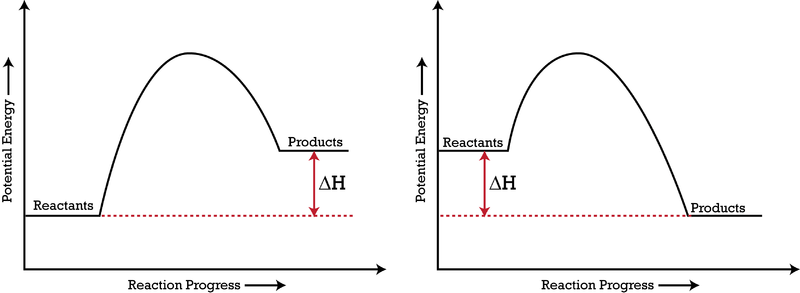Figure $$\PageIndex{3}$$: A potential energy diagram shows the total potential energy of a reacting system as the reaction proceeds. (Left) In an endothermic reaction, the energy of the products is greater than the energy of the reactants, and $$\Delta H$$ is positive. (Right) In an exothermic reaction, the energy of the products is lower than the energy of the reactants, and $$\Delta H$$ is negative.

The activation energy for a reaction is illustrated in the potential energy diagram by the height of the hill between the reactants and the products. For this reason, the activation energy of a reaction is sometimes referred to as the activation energy barrier. Reacting particles must have enough energy so that when they collide, they can overcome this barrier (see figure below).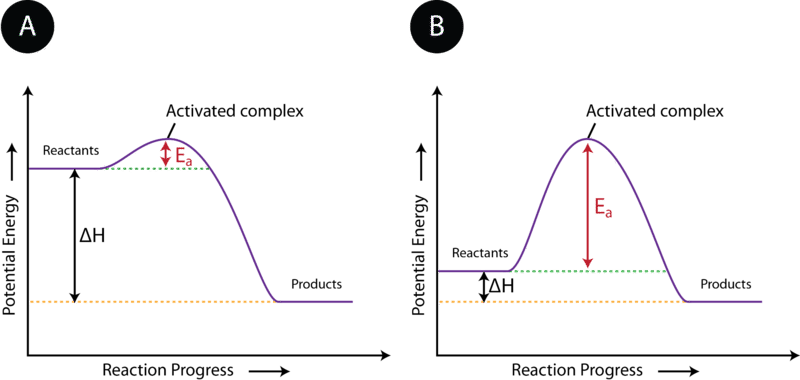Figure $$\PageIndex{4}$$: The activation energy $$\left( E_a \right)$$ of a reaction is the barrier that must be overcome in order for the reactants to become products. (A) The activation energy is low, meaning that the reaction is likely to be fast. (B) The activation energy is high, meaning that the reaction is likely to be slow.

As discussed earlier, reactant particles sometimes collide with one another and yet remain unchanged by the collision. Other times, the collision leads to the formation of products. The state of the particles that is in between the reactants and products is called the activated complex. An activated complex is an unstable arrangement of atoms that exists momentarily at the peak of the activation energy barrier. Because of its high energy, the activated complex exists only for an extremely short period of time (about $$10^{-13} \: \text{s}$$). The activated complex is equally likely to either reform the original reactants or go on to form the products. The figure below shows the formation of a possible activated complex between colliding hydrogen and oxygen molecules. Because of their unstable nature and brief existence, very little is known about the exact structures of most activated complexes.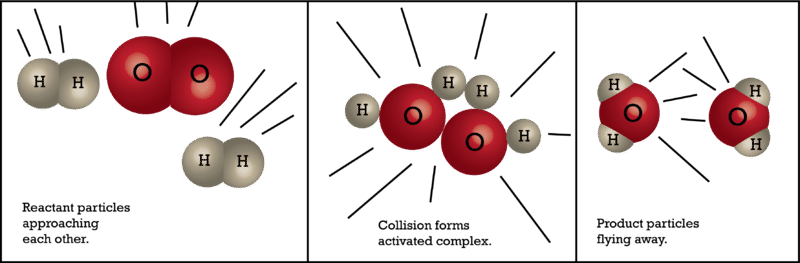Figure $$\PageIndex{5}$$: An activated complex is a short-lived state in which the colliding particles are at the peak of the potential energy curve.

Factors Affecting Reaction Rates

By their nature, some reactions occur very quickly, while others are very slow. However, certain changes in the reaction conditions can have an effect on the rate of a given chemical reaction. Collision theory can be utilized to explain these rate effects.

Concentration

Increasing the concentration of one or more of the reacting substances generally increases the reaction rate. When more particles are present in a given amount of space, a greater number of collisions will naturally occur between those particles. Since the rate of a reaction is dependent on the frequency of collisions between the reactants, the rate increases as the concentration increases.

Pressure

When the pressure of a gas is increased, its particles are forced closer together, decreasing the amount of empty space between them. Therefore, an increase in the pressure of a gas is also an increase in the concentration of the gas. For gaseous reactions, an increase in pressure increases the rate of reaction for the same reasons as described above for an increase in concentration. Higher gas pressure leads to a greater frequency of collisions between reacting particles.

Surface Area

A large log placed in a fire will burn relatively slowly. If the same mass of wood were added to the fire in the form of small twigs, they would burn much more quickly. This is because the twigs provide a greater surface are than the log does. An increase in the surface area of a reactant increases the rate of a reaction. Surface area is larger when a given amount of a solid is present as smaller particles. A powdered reactant has a greater surface area than the same reactant as a solid chunk. In order to increase the surface area of a substance, it may be ground into smaller particles or dissolved into a liquid. In solution, the dissolved particles are separated from each other and will react more quickly with other reactants. The figure below shows the unfortunate result of high surface area in an unwanted combustion reaction. Small particles of grain dust are very susceptible to rapid reactions with oxygen, which can result in violent explosions and quick-burning fires.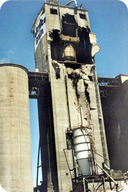Figure $$\PageIndex{6}$$: This grain elevator in Kansas exploded in 1998. The tiny size of the reacting particles (grain dust) caused the reaction with oxygen in the air to be violently explosive.

Temperature

Raising the temperature of a chemical reaction results in a higher reaction rate. When the reactant particles are heated, they move faster and faster, resulting in a greater frequency of collisions. An even more important effect of the temperature increase is that the collisions occur with a greater force, which means the reactants are more likely to surmount the activation energy barrier and go on to form products. Increasing the temperature of a reaction increases not only the frequency of collisions, but also the percentage of those collisions that are effective, resulting in an increased reaction rate.

Paper is certainly a highly combustible material, but paper does not burn at room temperature because the activation energy for the reaction is too high. The vast majority of collisions between oxygen molecules and the paper are ineffective. However, when the paper is heated by the flame from a match, it reaches a point where the molecules now have enough energy to react. The reaction is very exothermic, so the heat released by the initial reaction will provide enough energy to allow the reaction to continue, even if the match is removed. The paper continues to burn rapidly until it is gone.

Catalysts

The rates of some chemical reactions can be increased dramatically by introducing certain other substances into the reaction mixture. Hydrogen peroxide is used as a disinfectant for scrapes and cuts, and it can be found in many medicine cabinets as a $$3\%$$ aqueous solution. Hydrogen peroxide naturally decomposes to produce water and oxygen gas, but the reaction is very slow. A bottle of hydrogen peroxide will last for several years before it needs to be replaced. However, the addition of just a small amount of manganese (IV) oxide to hydrogen peroxide will cause it to decompose completely in just a matter of minutes. A catalyst is a substance that increases the rate of a chemical reaction without being used up in the reaction. It accomplishes this task by providing an alternate reaction pathway that has a lower activation energy barrier. After the reaction occurs, a catalyst returns to its original state, so catalysts can be used over and over again. Because it is neither a reactant nor a product, a catalyst is shown in a chemical equation by being written above the yield arrow.

$2 \ce{H_2O_2} \left( aq \right) \overset{\ce{MnO_2}}{\rightarrow} 2 \ce{H_2O} \left( l \right) + \ce{O_2} \left( g \right)$

A catalyst works by changing the mechanism of the reaction, which can be though of as the specific set of smaller steps by which the reactants become products. The important point is that the use of a catalyst lowers the overall activation energy of the reaction (see figure below). With a lower activation energy barrier, a greater percentage of reactant molecules are able to have effective collisions, and the reaction rate increases.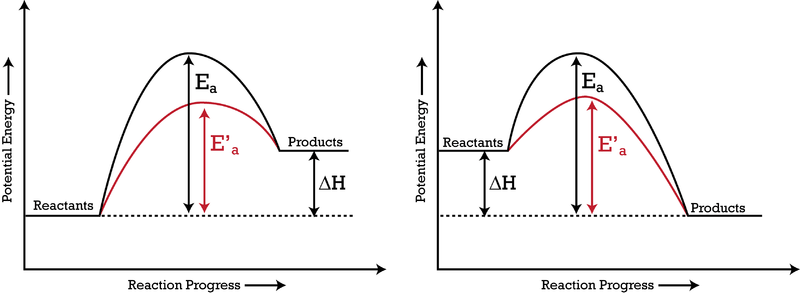Figure $$\PageIndex{7}$$: The addition of a catalyst to a reaction lowers the activation energy, increasing the rate of the reaction. The activation energy of the uncatalyzed reaction is shown by $$E_a$$, while the catalyzed reaction is shown by $$E_a'$$. The heat of reaction $$\left( \Delta H \right)$$ is unchanged by the presence of the catalyst.

Catalysts are extremely important parts of many chemical reactions. Enzymes in your body act as nature's catalysts, allowing important biochemical reactions to occur at reasonable rates. Chemical companies constantly search for new and better catalysts to make reactions go faster and thus make the company more profitable.